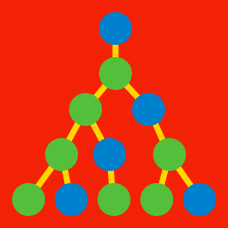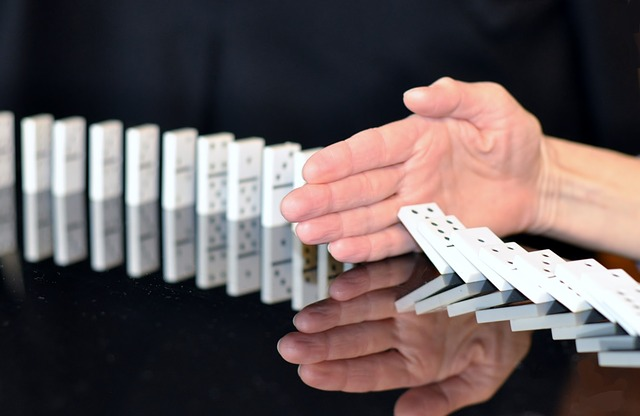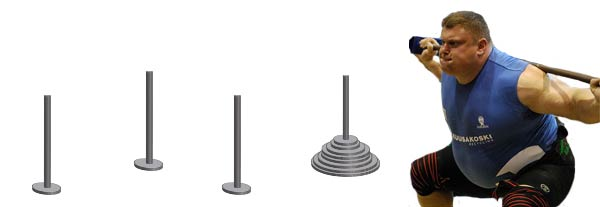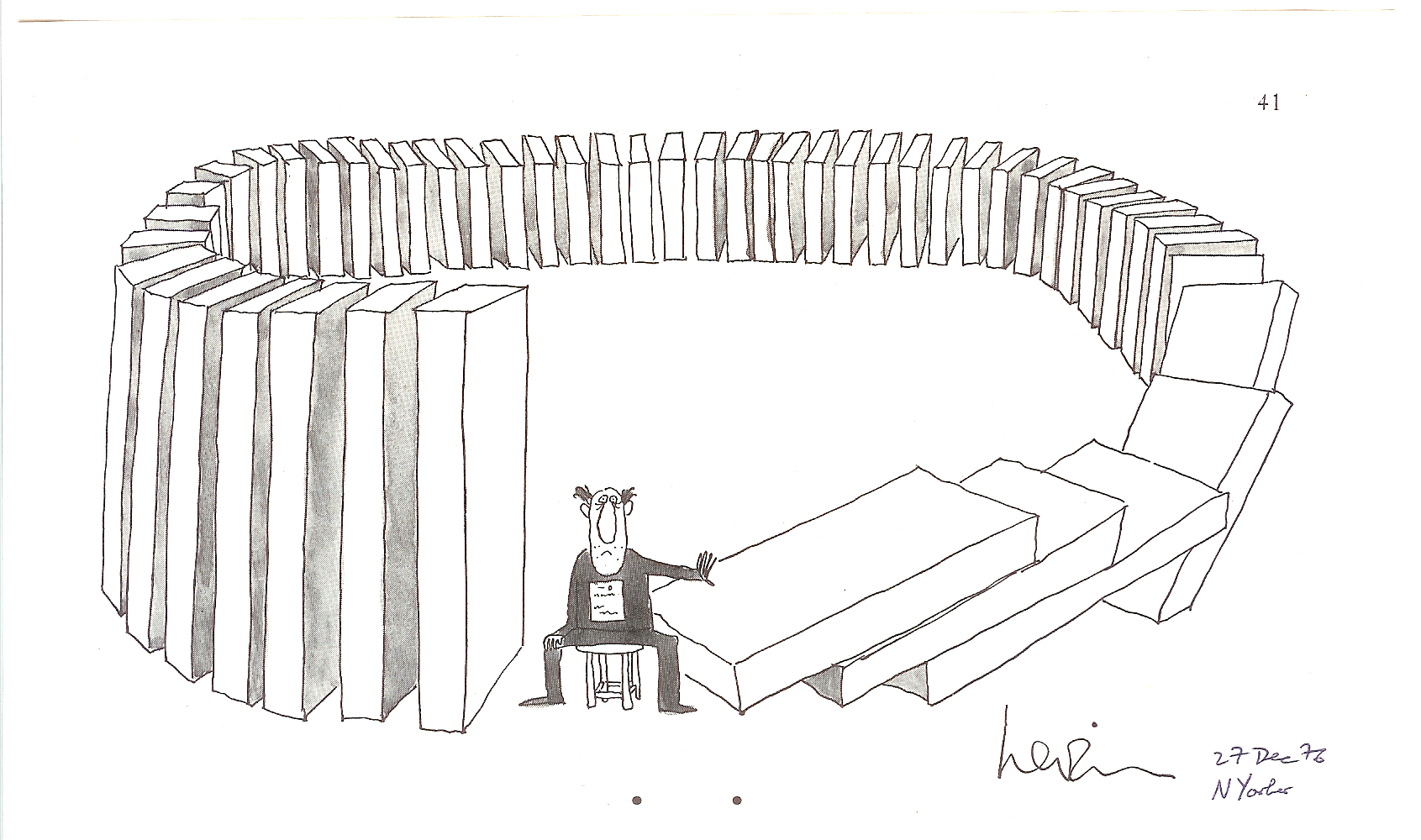Discrete Mathematics

Linear Recurrence Relations: Level 3 ChallengesA "domino" is a 1 by 2 or a 2 by 1 rectangle.
A 'domino tiling' of a region of the plane is a way of covering it (and only it) completely by non-overlapping dominoes. For example: There's 1 domino tiling of a 2 by1 rectangle & 2 tilings of a 2 by 2 rectangle. (1 consisting of 2 horizontal dominoes & 1 containing of 2 vertical ones) How many domino tilings of a 2 by 10 rectangle are there?At Walter's weight lifting gym there is a stack of 8 weight plates on a pole on the floor, along with 3 empty poles. Walter's physical training regimen involves moving the plates, one at a time, from one pole to another, making sure that a larger plate is never placed on top of a smaller plates.

Walter will end his workout once all of the plates have been moved off of the starting pole and are all on the same pole. Today, Walter is feeling lazy, so he wants to do this in as few moves as possible. What is the minimum number of moves that Walter must make in order to finish his workout?

Details and assumptions

If you are interested in stacking problems, you might want to look at This Robotic Arm Can Do Many Things, But Is Unable To Think For Itself$(\alpha_n)$ and $(\beta_n)$ are two sequences defined recursively as $\alpha_n=2 \alpha_{n-1}+1$ and $\beta_n=8 \beta_{n-1}+1$ with $\alpha_0=\beta_0=0.$ Find the ratio

$\frac{\alpha_{2016}}{\beta_{672}}.$

Consider a sequence $\{a_i\}$ of positive integers defined by $a_1= 1, a_2= 2$, and for all integers $n>2$, $a_n= 3a_{n-1} + 5a_{n-2}$ Consider the set $S= \{a_1, a_2, \cdots , a_{1200} \}$ Sam randomly picks an element from this set. The probability that this element is a multiple of $8$ can be expressed as $\dfrac{a}{b}$, where $a, b$ are coprime positive integers. Find $a+b$.

Define $X_n=X_{n-1}+X_{n-2}$

If $X_1=5^5$ and $X_2=5^6$

Find $\displaystyle \lim_{n\rightarrow \infty} \dfrac{X_{n+1}}{X_{n}}$

×